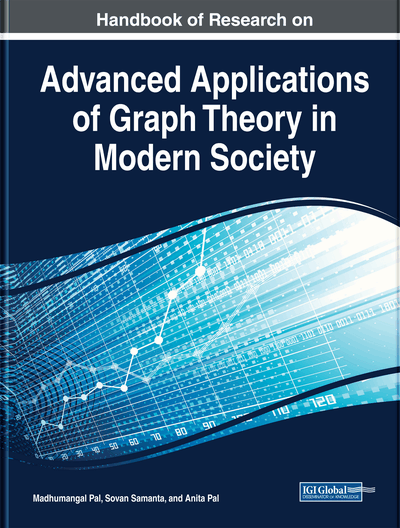# A Novel Weighted First Zagreb Index of Graph

Jibonjyoti Buragohain (Dibrugarh University, India) and A. Bharali (Dibrugarh University, India)
DOI: 10.4018/978-1-5225-9380-5.ch004
Available
\$37.50
No Current Special Offers

## Abstract

The Zagreb indices are the oldest among all degree-based topological indices. For a connected graph G, the first Zagreb index M1(G) is the sum of the term dG(u)+dG(v) corresponding to each edge uv in G, that is, M1 , where dG(u) is degree of the vertex u in G. In this chapter, the authors propose a weighted first Zagreb index and calculate its values for some standard graphs. Also, the authors study its correlations with various physico-chemical properties of octane isomers. It is found that this novel index has strong correlation with acentric factor and entropy of octane isomers as compared to other existing topological indices.
Chapter Preview
Top

## Introduction

The introduction of topological index or molecular structure descriptor in literature is a great success as it can correlate various physical properties, biological reactivity or chemical activity of molecules without undergoing actual experimentation. A topological index is a real number that can be associated to a molecule based on its molecular graph. A molecular graph is a simple graph corresponding to a molecule in which vertices represents atoms and edges represents various chemical bonds between them. For historical background of the topological index interested reader can go through (Trinajstić, 2011). The success of this simple mathematical quantity is because of the fact that the properties of a chemical compound is highly related to its molecular structure and we calculate the indices directly from the molecular graph of a molecule using various graph theoretic notions (e.g. degree, distance, etc). Some of the topological indices may be found in (Das & Tinajstić, 2010; Gutman, 2013; Estrada, 2000; Gutman, Milovanović & et al., 2018) and the references therein.

## Complete Chapter List

Search this Book:
Reset